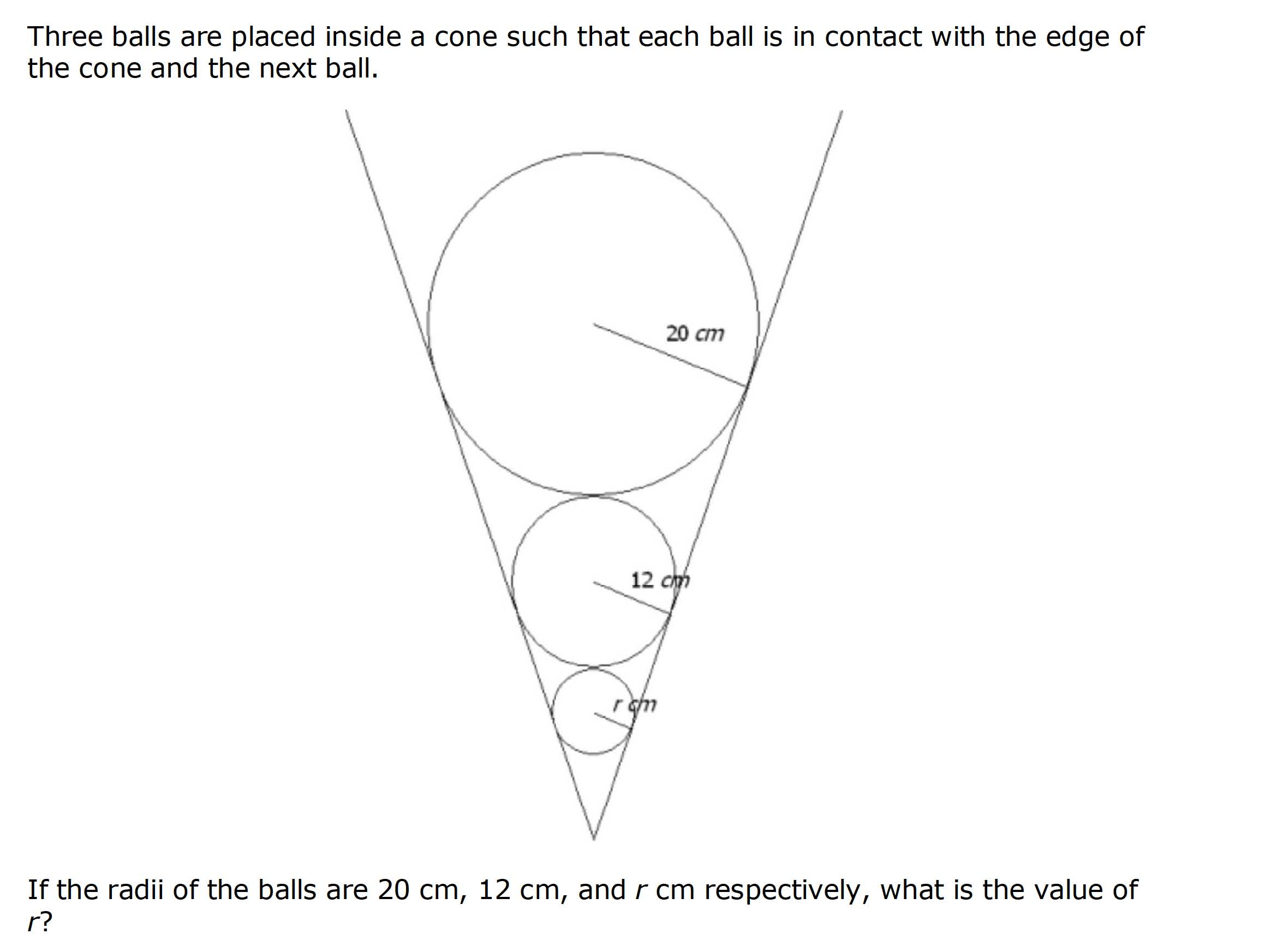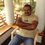# Problem of this dayFind the radius of the smaller circle.Note by Indulal Gopal
6 years, 9 months ago

This discussion board is a place to discuss our Daily Challenges and the math and science related to those challenges. Explanations are more than just a solution — they should explain the steps and thinking strategies that you used to obtain the solution. Comments should further the discussion of math and science.

When posting on Brilliant:

• Use the emojis to react to an explanation, whether you're congratulating a job well done , or just really confused .
• Ask specific questions about the challenge or the steps in somebody's explanation. Well-posed questions can add a lot to the discussion, but posting "I don't understand!" doesn't help anyone.
• Try to contribute something new to the discussion, whether it is an extension, generalization or other idea related to the challenge.

MarkdownAppears as
*italics* or _italics_ italics
**bold** or __bold__ bold
- bulleted- list
• bulleted
• list
1. numbered2. list
1. numbered
2. list
Note: you must add a full line of space before and after lists for them to show up correctly
paragraph 1paragraph 2

paragraph 1

paragraph 2

[example link](https://brilliant.org)example link
> This is a quote
This is a quote
    # I indented these lines
# 4 spaces, and now they show
# up as a code block.

print "hello world"
# I indented these lines
# 4 spaces, and now they show
# up as a code block.

print "hello world"
MathAppears as
Remember to wrap math in $$ ... $$ or $ ... $ to ensure proper formatting.
2 \times 3 $2 \times 3$
2^{34} $2^{34}$
a_{i-1} $a_{i-1}$
\frac{2}{3} $\frac{2}{3}$
\sqrt{2} $\sqrt{2}$
\sum_{i=1}^3 $\sum_{i=1}^3$
\sin \theta $\sin \theta$
\boxed{123} $\boxed{123}$

Sort by:

Using Basic proportionality theorem $\frac{r}{12} = \frac{12}{20} \Rightarrow r = \frac{144}{20} = \boxed{7.2}$

- 6 years, 9 months ago

Once you find the like triangles it's not that hard anymore :)

- 6 years, 9 months ago

exactly

- 6 years, 9 months ago

I converted it into a problem of Straight Lines. Mapping the distance of the centre of a sphere from the topmost point on the y axis , and radius on the x axis, I calculated slope by calculating dy/dx of the given 2 spheres as (52-20)/(12-20) = -4 . Then using the equation of straight line y=mx+c and putting the value of y=52 and x=12, 52=(-4)x12 +c, c comes out to be 100. Then for the unknown radius , we know y=64+r and x=r. Solving this 64+r=(-4) x r +100 which gives r=7.2........ Cool approach, isn't it?

- 6 years, 9 months ago

but just have a little hard to understand your formula...

- 6 years, 9 months ago

r = 7.2cm

- 6 years, 9 months ago

Salman , I think you made an error. Its not 5 cm. Think and do. You can solve it

- 6 years, 9 months ago

N ths is a prblm -_- Simply,36/5 gives 7.2

- 6 years, 9 months ago

Where'd you get 36 and 5?

- 6 years, 9 months ago

1.2

- 6 years, 9 months ago

Nope :)

- 6 years, 9 months ago

7.2 cm.

- 6 years, 9 months ago

7.2 cm

- 6 years, 9 months ago

- 6 years, 9 months ago

- 6 years, 9 months ago

...Well... $\dfrac{12}{20}=\dfrac{x}{12}\Rightarrow x=\boxed{\dfrac{36}{5}}$. Waaay too simple.

- 6 years, 9 months ago

but how do you get 36/5 ?

- 6 years, 9 months ago

From above we can get $x = \frac{144}{20} = \frac{36}{5}$

- 6 years, 9 months ago

7.2

- 6 years, 9 months ago

7.2 cm

- 6 years, 9 months ago

7.2

- 6 years, 9 months ago

7.2cm?? or 4.0cm??

- 6 years, 9 months ago

7.2 cm

- 6 years, 9 months ago

7.2 cm

- 6 years, 9 months ago

7.2 cm...

- 6 years, 9 months ago

7.2 cm

- 6 years, 9 months ago

I think the proper answer is 7.2cm if I no mistaken...

- 6 years, 9 months ago

7.2

- 6 years, 9 months ago

3

- 6 years, 9 months ago

7.2 cm

- 6 years, 9 months ago

7.2

- 6 years, 9 months ago

It is too easy to be the problem of the day .r=7.2cm

- 6 years, 9 months ago

7.2

- 6 years, 9 months ago

use basic proportionality theorem r/12=12/20 r=7.2

- 6 years, 8 months ago

This problem can be resolved by using Geometric progression. The rays are in G.P. . So 20/12=5/3 it's the common ratio. 12/5/3= 7,2=r

- 6 years, 8 months ago

Similar triangles

7.2 cm

- 6 years, 8 months ago

20/12=12/r r=7.2

- 6 years, 1 month ago

4

- 6 years ago

72

- 6 years ago

7.2 cm

- 6 years ago

Just basic proportionality funda and you are through. r/12=12/20 r=7.2cm

- 6 years ago

20:12 = 5:3 12:r = 5:3 r = 3:5 x 12 r = 7,2 what a simple

- 4 years, 10 months ago

- 6 years, 9 months ago

No, it's not.

- 6 years, 9 months ago